# Selina Solutions Concise Mathematics Class 6 Chapter 32 Perimeter and Area of Plane Figures Exercise 32(B)

Selina Solutions Concise Mathematics Class 6 Chapter 32 Perimeter and Area of Plane Figures Exercise 32(B) are designed by the experts at BYJU’S to boost confidence among students. The important steps to be followed in determining the area of rectangle, square and triangle are explained in brief under this exercise. The solutions contain comprehensive answers to make learning fun for the students. Those who aim to speed up their problem solving skills are suggested to practice these solutions repeatedly. For a better hold on these concepts, students can follow Selina Solutions Concise Mathematics Class 6 Chapter 32 Perimeter and Area of Plane Figures Exercise 32(B) PDF, from the links which are available below.

## Selina Solutions Concise Mathematics Class 6 Chapter 32 Perimeter and Area of Plane Figures Exercise 32(B) Download PDF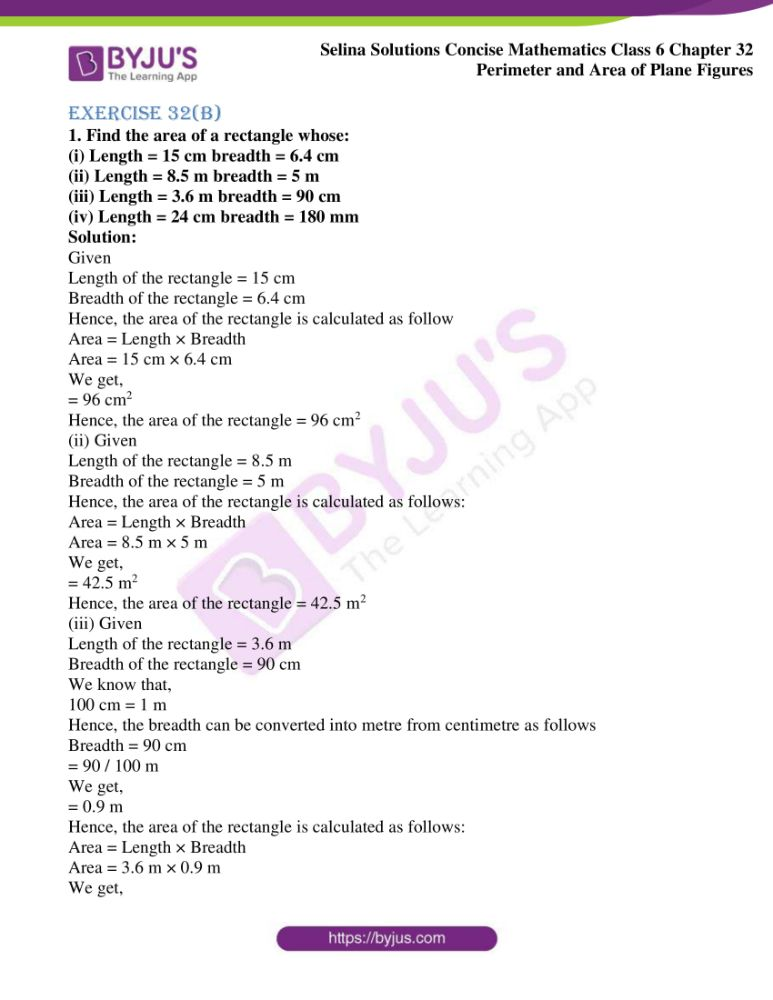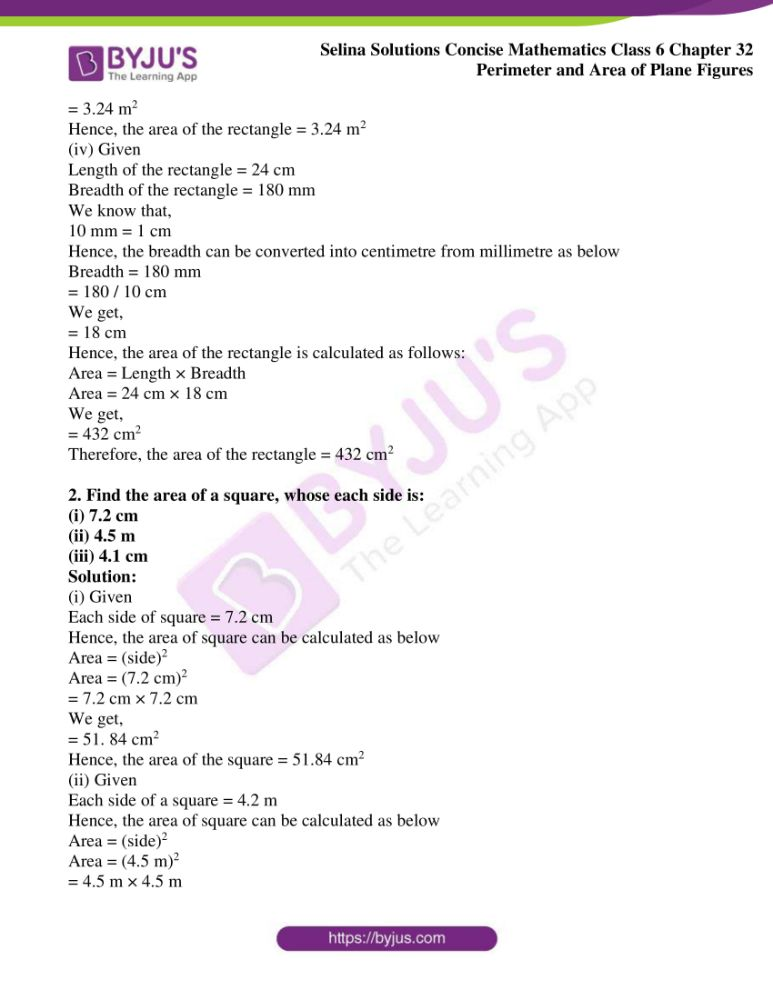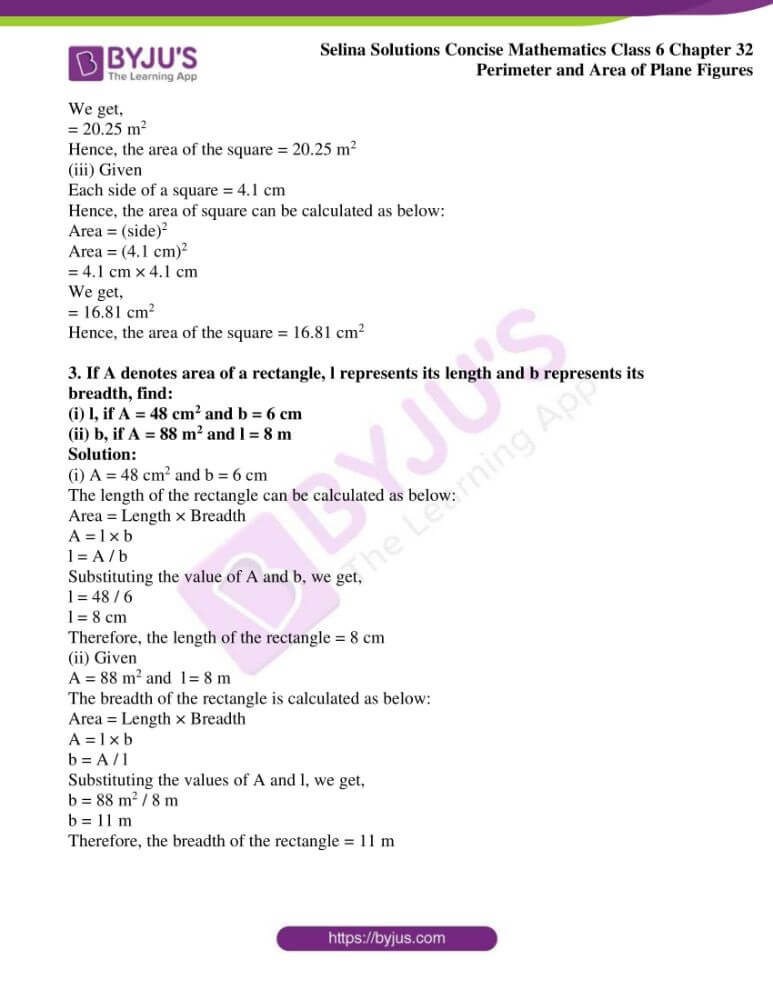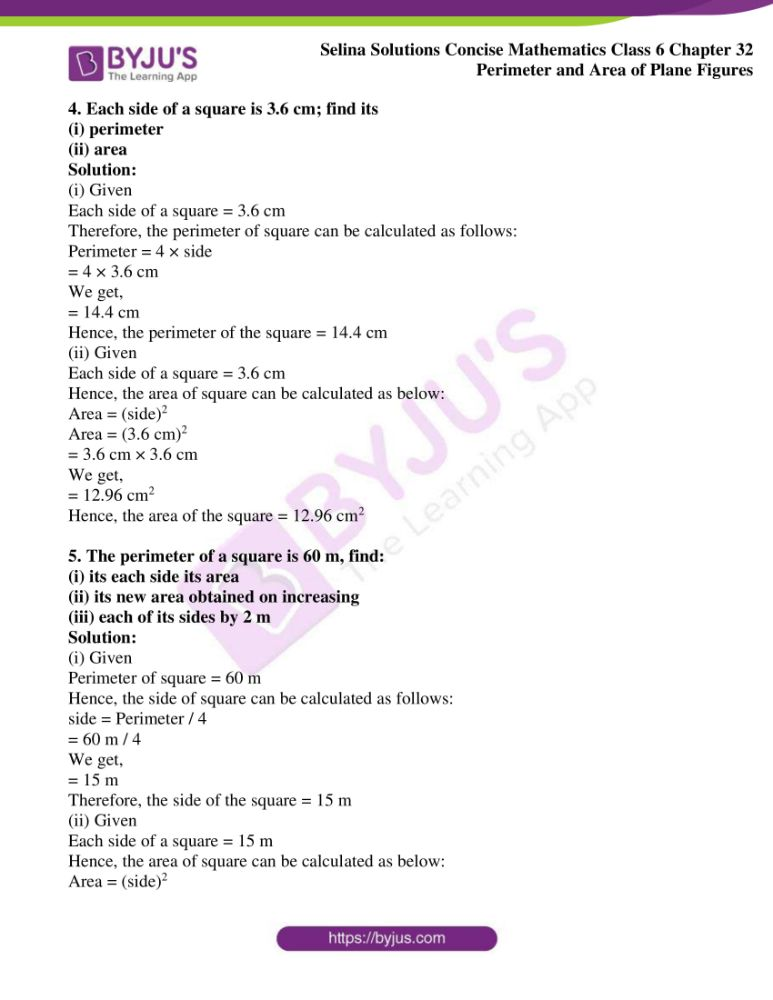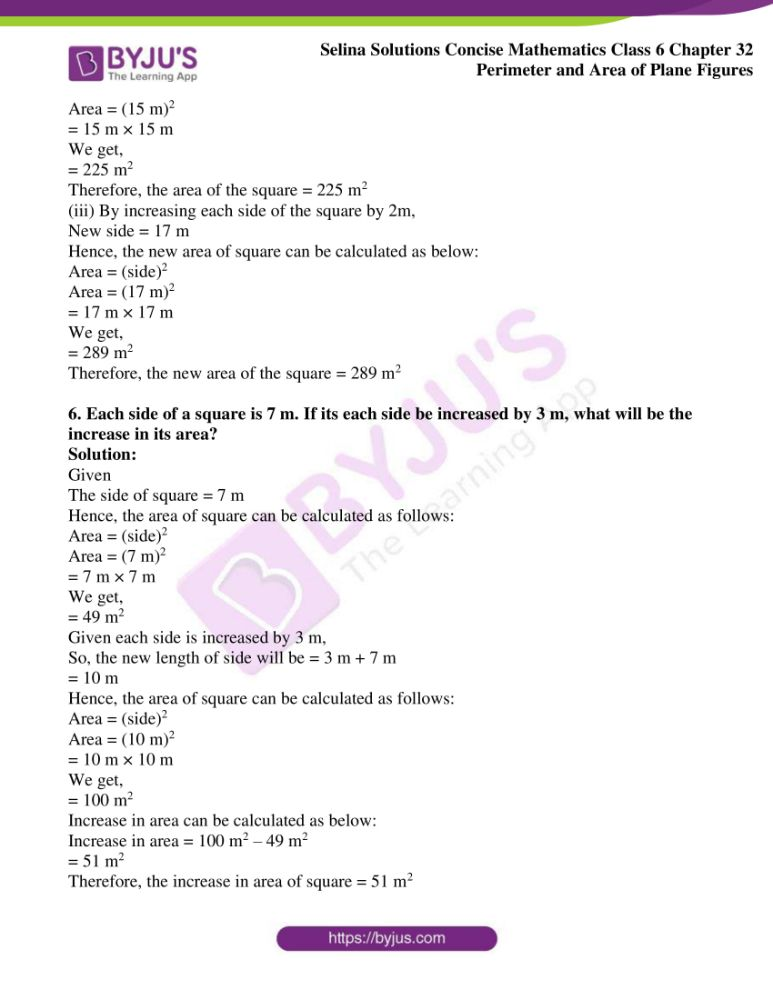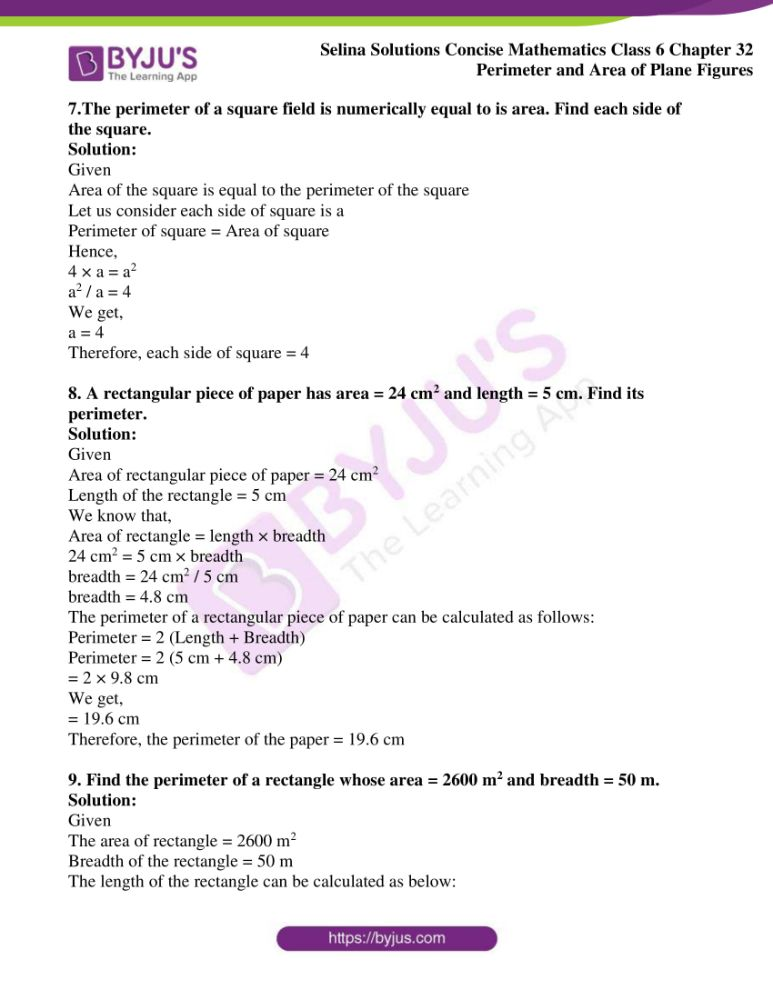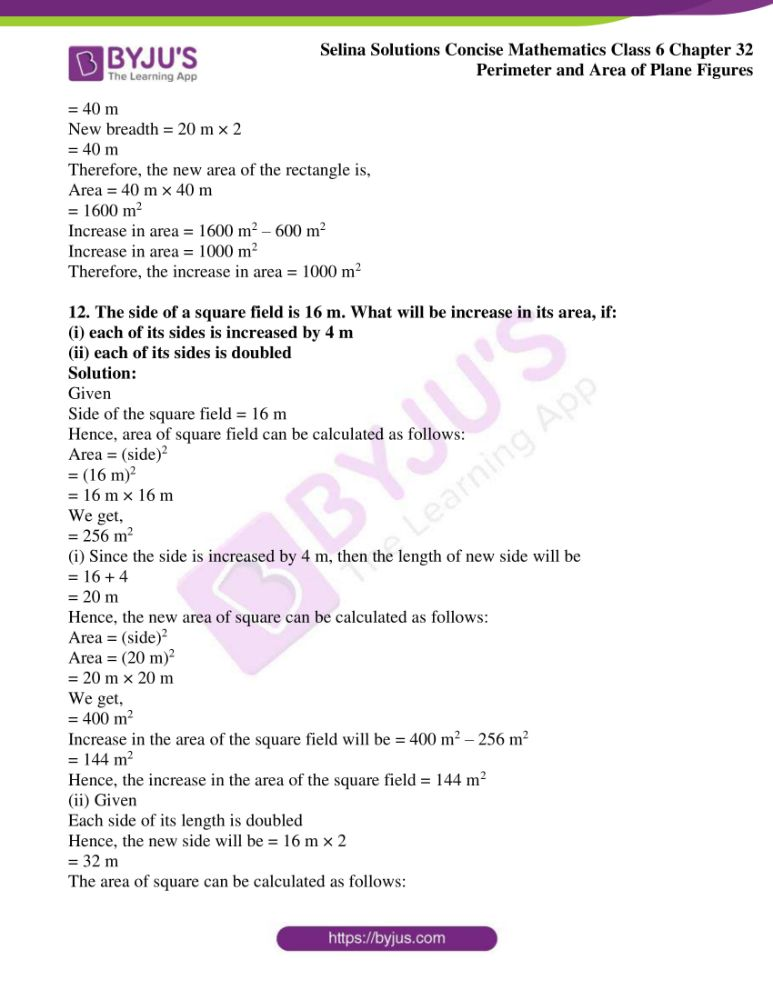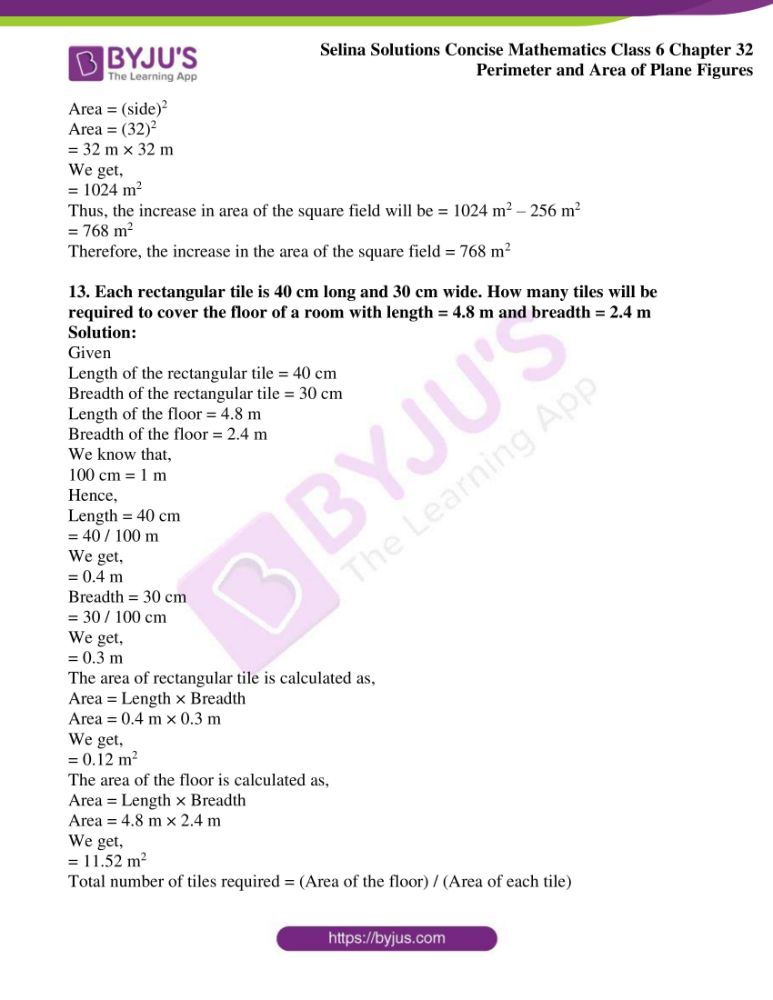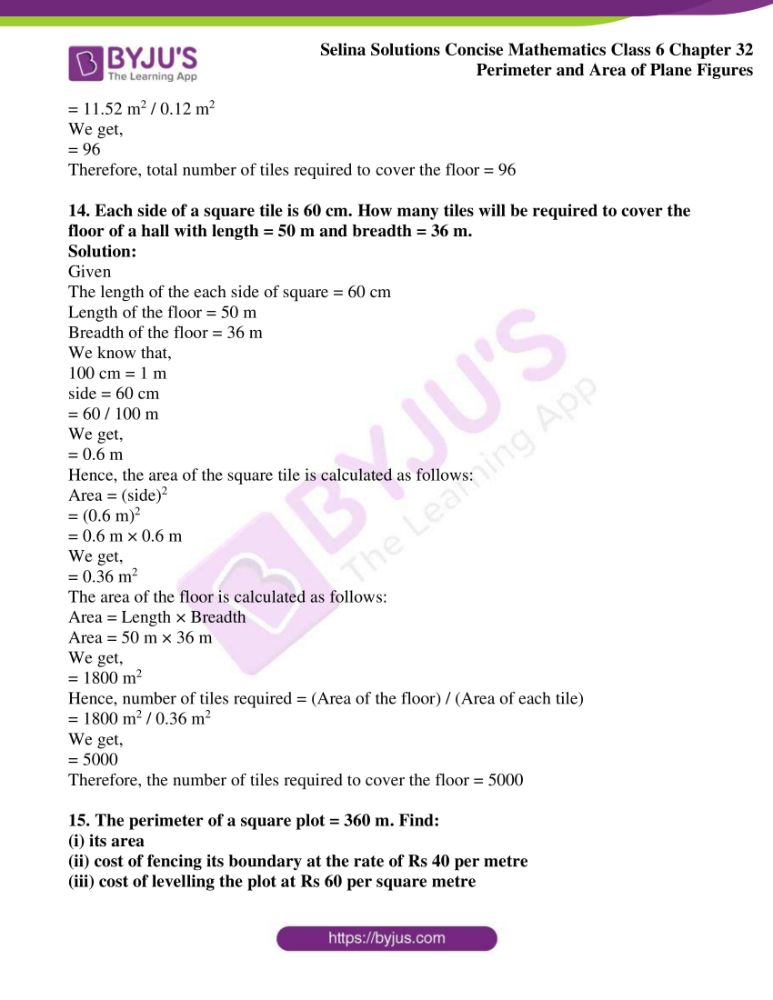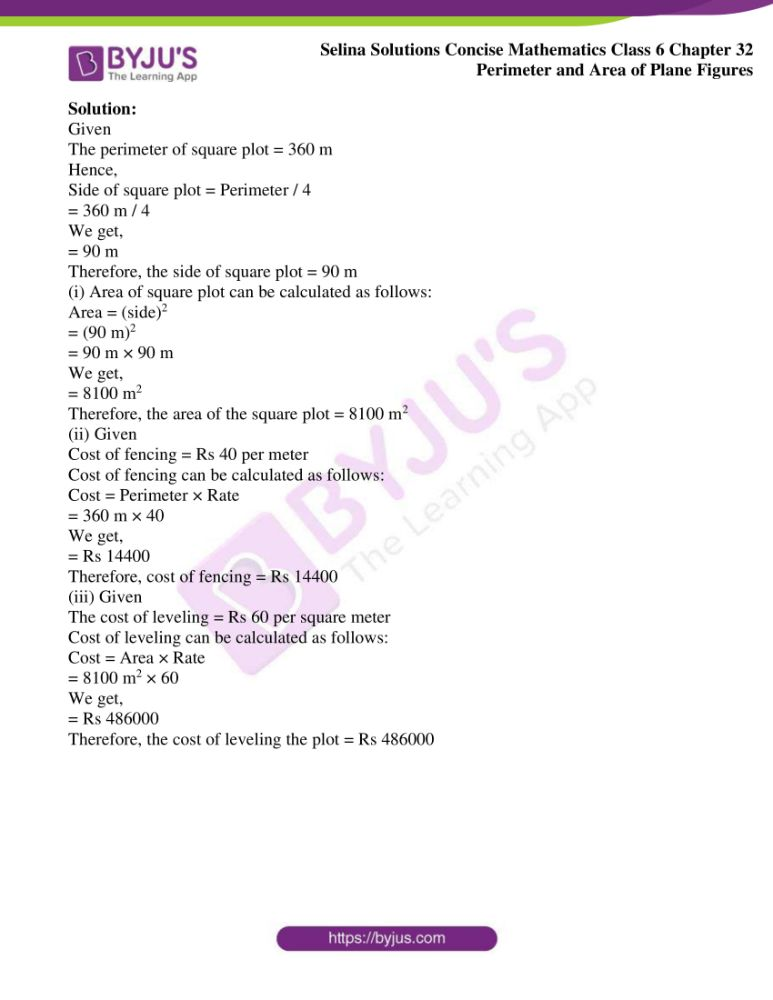### Access another exercise of Selina Solutions Concise Mathematics Class 6 Chapter 32 Perimeter and Area of Plane Figures

Exercise 32(A) Solutions

### Access Selina Solutions Concise Mathematics Class 6 Chapter 32 Perimeter and Area of Plane Figures Exercise 32(B)

Exercise 32(B)

1. Find the area of a rectangle whose:

(i) Length = 15 cm breadth = 6.4 cm

(ii) Length = 8.5 m breadth = 5 m

(iii) Length = 3.6 m breadth = 90 cm

(iv) Length = 24 cm breadth = 180 mm

Solution:

Given

Length of the rectangle = 15 cm

Breadth of the rectangle = 6.4 cm

Hence, the area of the rectangle is calculated as follow

Area = 15 cm × 6.4 cm

We get,

= 96 cm2

Hence, the area of the rectangle = 96 cm2

(ii) Given

Length of the rectangle = 8.5 m

Breadth of the rectangle = 5 m

Hence, the area of the rectangle is calculated as follows:

Area = 8.5 m × 5 m

We get,

= 42.5 m2

Hence, the area of the rectangle = 42.5 m2

(iii) Given

Length of the rectangle = 3.6 m

Breadth of the rectangle = 90 cm

We know that,

100 cm = 1 m

Hence, the breadth can be converted into metre from centimetre as follows

= 90 / 100 m

We get,

= 0.9 m

Hence, the area of the rectangle is calculated as follows:

Area = 3.6 m × 0.9 m

We get,

= 3.24 m2

Hence, the area of the rectangle = 3.24 m2

(iv) Given

Length of the rectangle = 24 cm

Breadth of the rectangle = 180 mm

We know that,

10 mm = 1 cm

Hence, the breadth can be converted into centimetre from millimetre as below

= 180 / 10 cm

We get,

= 18 cm

Hence, the area of the rectangle is calculated as follows:

Area = 24 cm × 18 cm

We get,

= 432 cm2

Therefore, the area of the rectangle = 432 cm2

2. Find the area of a square, whose each side is:

(i) 7.2 cm

(ii) 4.5 m

(iii) 4.1 cm

Solution:

(i) Given

Each side of square = 7.2 cm

Hence, the area of square can be calculated as below

Area = (side)2

Area = (7.2 cm)2

= 7.2 cm × 7.2 cm

We get,

= 51. 84 cm2

Hence, the area of the square = 51.84 cm2

(ii) Given

Each side of a square = 4.2 m

Hence, the area of square can be calculated as below

Area = (side)2

Area = (4.5 m)2

= 4.5 m × 4.5 m

We get,

= 20.25 m2

Hence, the area of the square = 20.25 m2

(iii) Given

Each side of a square = 4.1 cm

Hence, the area of square can be calculated as below:

Area = (side)2

Area = (4.1 cm)2

= 4.1 cm × 4.1 cm

We get,

= 16.81 cm2

Hence, the area of the square = 16.81 cm2

3. If A denotes area of a rectangle, l represents its length and b represents its breadth, find:

(i) l, if A = 48 cm2 and b = 6 cm

(ii) b, if A = 88 m2 and l = 8 m

Solution:

(i) A = 48 cm2 and b = 6 cm

The length of the rectangle can be calculated as below:

A = l × b

l = A / b

Substituting the value of A and b, we get,

l = 48 / 6

l = 8 cm

Therefore, the length of the rectangle = 8 cm

(ii) Given

A = 88 m2 and l = 8 m

The breadth of the rectangle is calculated as below:

A = l × b

b = A / l

Substituting the values of A and l, we get,

b = 88 m2 / 8 m

b = 11 m

Therefore, the breadth of the rectangle = 11 m

4. Each side of a square is 3.6 cm; find its

(i) perimeter

(ii) area

Solution:

(i) Given

Each side of a square = 3.6 cm

Therefore, the perimeter of square can be calculated as follows:

Perimeter = 4 × side

= 4 × 3.6 cm

We get,

= 14.4 cm

Hence, the perimeter of the square = 14.4 cm

(ii) Given

Each side of a square = 3.6 cm

Hence, the area of square can be calculated as below:

Area = (side)2

Area = (3.6 cm)2

= 3.6 cm × 3.6 cm

We get,

= 12.96 cm2

Hence, the area of the square = 12.96 cm2

5. The perimeter of a square is 60 m, find:

(i) its each side its area

(ii) its new area obtained on increasing

(iii) each of its sides by 2 m

Solution:

(i) Given

Perimeter of square = 60 m

Hence, the side of square can be calculated as follows:

side = Perimeter / 4

= 60 m / 4

We get,

= 15 m

Therefore, the side of the square = 15 m

(ii) Given

Each side of a square = 15 m

Hence, the area of square can be calculated as below:

Area = (side)2

Area = (15 m)2

= 15 m × 15 m

We get,

= 225 m2

Therefore, the area of the square = 225 m2

(iii) By increasing each side of the square by 2m,

New side = 17 m

Hence, the new area of square can be calculated as below:

Area = (side)2

Area = (17 m)2

= 17 m × 17 m

We get,

= 289 m2

Therefore, the new area of the square = 289 m2

6. Each side of a square is 7 m. If its each side be increased by 3 m, what will be the increase in its area?

Solution:

Given

The side of square = 7 m

Hence, the area of square can be calculated as follows:

Area = (side)2

Area = (7 m)2

= 7 m × 7 m

We get,

= 49 m2

Given each side is increased by 3 m,

So, the new length of side will be = 3 m + 7 m

= 10 m

Hence, the area of square can be calculated as follows:

Area = (side)2

Area = (10 m)2

= 10 m × 10 m

We get,

= 100 m2

Increase in area can be calculated as below:

Increase in area = 100 m2 – 49 m2

= 51 m2

Therefore, the increase in area of square = 51 m2

7.The perimeter of a square field is numerically equal to is area. Find each side of the square.

Solution:

Given

Area of the square is equal to the perimeter of the square

Let us consider each side of square is a

Perimeter of square = Area of square

Hence,

4 × a = a2

a2 / a = 4

We get,

a = 4

Therefore, each side of square = 4

8. A rectangular piece of paper has area = 24 cm2 and length = 5 cm. Find its perimeter.

Solution:

Given

Area of rectangular piece of paper = 24 cm2

Length of the rectangle = 5 cm

We know that,

Area of rectangle = length × breadth

24 cm2 = 5 cm × breadth

breadth = 24 cm2 / 5 cm

The perimeter of a rectangular piece of paper can be calculated as follows:

Perimeter = 2 (Length + Breadth)

Perimeter = 2 (5 cm + 4.8 cm)

= 2 × 9.8 cm

We get,

= 19.6 cm

Therefore, the perimeter of the paper = 19.6 cm

9. Find the perimeter of a rectangle whose area = 2600 m2 and breadth = 50 m.

Solution:

Given

The area of rectangle = 2600 m2

Breadth of the rectangle = 50 m

The length of the rectangle can be calculated as below:

= 2600 m2 / 50 m

We get,

= 52 m

Hence, the perimeter of rectangle can be calculated as below:

Perimeter = 2 (Length + Breadth)

Perimeter = 2 (52 m + 50 m)

= 2 × 102 m

We get,

= 204 m

Therefore, the perimeter of the rectangle = 204 m

10. What will happen to the area of a rectangle, if its length and breadth both are trippled?

Solution:

Let us consider the length of the rectangle is l and breadth of the rectangle is b

Since,

Length and breadth of the rectangle are trippled

So, new length and breadth will be = 3l × 3b

Hence, the new area of the rectangle is,

Area = 3l × 3b

We get,

Area = 9 × l × b

Therefore, the new area will be 9 times than the original area of the rectangle

11. Length of a rectangle is 30 m and its breadth is 20 m. Find the increase in its area if its length is increased by 10 m and its breadth is doubled.

Solution:

Given

The length of a rectangle = 30 m

The breadth of rectangle = 20 m

The area of the rectangle can be calculated as follows:

= 30 m × 20 m

= 600 m2

Also, given that the length is increased by 10 m and its breadth is doubled

Hence, the new length and breadth is as follows:

New length = 30 m + 10 m

= 40 m

New breadth = 20 m × 2

= 40 m

Therefore, the new area of the rectangle is,

Area = 40 m × 40 m

= 1600 m2

Increase in area = 1600 m2 – 600 m2

Increase in area = 1000 m2

Therefore, the increase in area = 1000 m2

12. The side of a square field is 16 m. What will be increase in its area, if:

(i) each of its sides is increased by 4 m

(ii) each of its sides is doubled

Solution:

Given

Side of the square field = 16 m

Hence, area of square field can be calculated as follows:

Area = (side)2

= (16 m)2

= 16 m × 16 m

We get,

= 256 m2

(i) Since the side is increased by 4 m, then the length of new side will be

= 16 + 4

= 20 m

Hence, the new area of square can be calculated as follows:

Area = (side)2

Area = (20 m)2

= 20 m × 20 m

We get,

= 400 m2

Increase in the area of the square field will be = 400 m2 – 256 m2

= 144 m2

Hence, the increase in the area of the square field = 144 m2

(ii) Given

Each side of its length is doubled

Hence, the new side will be = 16 m × 2

= 32 m

The area of square can be calculated as follows:

Area = (side)2

Area = (32)2

= 32 m × 32 m

We get,

= 1024 m2

Thus, the increase in area of the square field will be = 1024 m2 – 256 m2

= 768 m2

Therefore, the increase in the area of the square field = 768 m2

13. Each rectangular tile is 40 cm long and 30 cm wide. How many tiles will be required to cover the floor of a room with length = 4.8 m and breadth = 2.4 m

Solution:

Given

Length of the rectangular tile = 40 cm

Breadth of the rectangular tile = 30 cm

Length of the floor = 4.8 m

Breadth of the floor = 2.4 m

We know that,

100 cm = 1 m

Hence,

Length = 40 cm

= 40 / 100 m

We get,

= 0.4 m

= 30 / 100 cm

We get,

= 0.3 m

The area of rectangular tile is calculated as,

Area = 0.4 m × 0.3 m

We get,

= 0.12 m2

The area of the floor is calculated as,

Area = 4.8 m × 2.4 m

We get,

= 11.52 m2

Total number of tiles required = (Area of the floor) / (Area of each tile)

= 11.52 m2 / 0.12 m2

We get,

= 96

Therefore, total number of tiles required to cover the floor = 96

14. Each side of a square tile is 60 cm. How many tiles will be required to cover the floor of a hall with length = 50 m and breadth = 36 m.

Solution:

Given

The length of the each side of square = 60 cm

Length of the floor = 50 m

Breadth of the floor = 36 m

We know that,

100 cm = 1 m

side = 60 cm

= 60 / 100 m

We get,

= 0.6 m

Hence, the area of the square tile is calculated as follows:

Area = (side)2

= (0.6 m)2

= 0.6 m × 0.6 m

We get,

= 0.36 m2

The area of the floor is calculated as follows:

Area = 50 m × 36 m

We get,

= 1800 m2

Hence, number of tiles required = (Area of the floor) / (Area of each tile)

= 1800 m2 / 0.36 m2

We get,

= 5000

Therefore, the number of tiles required to cover the floor = 5000

15. The perimeter of a square plot = 360 m. Find:

(i) its area

(ii) cost of fencing its boundary at the rate of Rs 40 per metre

(iii) cost of levelling the plot at Rs 60 per square metre

Solution:

Given

The perimeter of square plot = 360 m

Hence,

Side of square plot = Perimeter / 4

= 360 m / 4

We get,

= 90 m

Therefore, the side of square plot = 90 m

(i) Area of square plot can be calculated as follows:

Area = (side)2

= (90 m)2

= 90 m × 90 m

We get,

= 8100 m2

Therefore, the area of the square plot = 8100 m2

(ii) Given

Cost of fencing = Rs 40 per meter

Cost of fencing can be calculated as follows:

Cost = Perimeter × Rate

= 360 m × 40

We get,

= Rs 14400

Therefore, cost of fencing = Rs 14400

(iii) Given

The cost of leveling = Rs 60 per square meter

Cost of leveling can be calculated as follows:

Cost = Area × Rate

= 8100 m2 × 60

We get,

= Rs 486000

Therefore, the cost of leveling the plot = Rs 486000